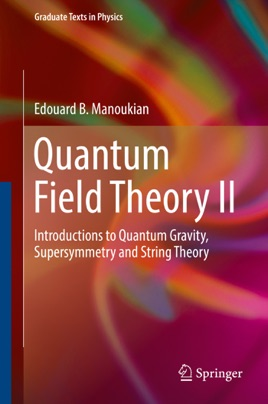• USD 54.99

## Descripción de editorial

This book takes a pedagogical approach to explaining quantum gravity, supersymmetry and string theory in a coherent way. It is aimed at graduate students and researchers in quantum field theory and high-energy physics.

The first part of the book introduces quantum gravity, without requiring previous knowledge of general relativity (GR). The necessary geometrical aspects are derived afresh leading to explicit general Lagrangians for gravity, including that of general relativity. The quantum aspect of gravitation, as described by the graviton, is introduced and perturbative quantum GR is discussed. The Schwinger-DeWitt formalism is developed to compute the one-loop contribution to the theory and renormalizability aspects of the perturbative theory are also discussed. This follows by introducing only the very basics of a non-perturbative, background-independent, formulation of quantum gravity, referred to as “loop quantum gravity”, which gives rise to a quantization of space.

In the second part the author introduces supersymmetry and its consequences. The generation of superfields is represented in detail.  Supersymmetric generalizations of Maxwell’s Theory as well as of Yang-Mills field theory, and of the standard model are worked out. Spontaneous symmetry breaking, improvement of the divergence problem in supersymmetric field theory, and its role in the hierarchy problem are covered. The unification of the fundamental constants in a supersymmetric version of the standard model are then studied. Geometrical aspects necessary to study supergravity are developed culminating in the derivation of its full action.

The third part introduces string theory and the analysis of the spectra of the mass (squared) operator associated with the oscillating strings. The properties of the underlying fields, associated with massless particles, encountered in string theory are studied in some detail. Elements of compactification, duality and D-branes are given, as well of the generation of vertices and interactions of strings. In the final sections, the author shows how to recover GR and the Yang-Mills field Theory from string theory.